# Exploring IBM HR data using Python

## Intro

This tutorial series explores the IBM HR data set. This data is typically used to demonstrate the ability of various machine learning algorithms applied to HR data.

In this series, I'll use it to demonstrate the awesome power Python can bring to HR data

Sections

• Statistics
• Matplotlib
• Pandas
• Seaborn
• Plotly
• Findings
In :
__author__ = "adam"
__version__ = "1.0.0"

In :
# imports
import numpy as np
import pandas as pd

import matplotlib.pyplot as plt

%matplotlib inline

In :
# if continuing on from the previous section, read the data from saved file


In :
# read the data directly from IBM Watson Analytics
# using pandas read excel file into dataframe

# save data for later
# empl_data.to_excel("WA_Fn-UseC_-HR-Employee-Attrition.xlsx")


## matplotlib

Matplotlib is the backbone of plotting in Python. If you're familiar with R, think Base Graphics. They're similar in that they are powerful alone, but become even more powerful when higher-level packages build on top of these respective base packages.

As Matplotlib is the base of many higher-level Python plotting packages, we'll start our EDA with it.

As you'll see next, it's very easy to get started. And, as we've loaded our data into a DataFrame, it's easy to pass the information we want to Matplotlib using techniques we learned briefly in the first seciton of this series.

We'll skip all of the statistics and get right into visualizations, where we'll explore the 'Age' dimension of our data.

Now, show me the data!

In :
# first, determine the average age
avg_age = empl_data['Age'].mean()
avg_age

Out:
36.923809523809524
In :
# plot average age
plt.bar('Age',avg_age)

Out:
<BarContainer object of 1 artists>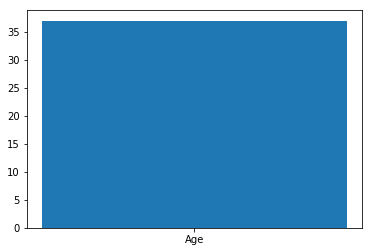With just a few short lines, we've been able to create a plot of the average age of employees

We can improve the readability of this plot by adding items such as the actual value and a title

In :
# average employee age

#create the figure
plt.figure(figsize=(4,4))

# calculate average age
avg_age = empl_data['Age'].mean()

# round avg_age for display
display_age = round(avg_age, 2)

# create the plot
plt.bar('Age', avg_age)

plt.text('Age', avg_age, display_age, va='bottom', ha='center')

plt.title('Average Employee Age')

# display the plot
plt.show()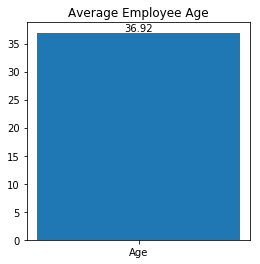With a few additional lines, we added a lot more clarity to the plot in order to share and communicate the information properly.

Next, let's look at the average age within each department.

In :
# average employee age by department

plt.figure(figsize=(9,4))
bars = plt.bar(empl_data['Department'].unique(),empl_data.groupby('Department')['Age'].mean())

plt.title('Average Age by Department')
plt.show()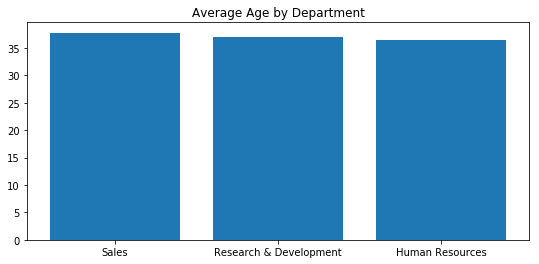The plot looks good, and gives us the insight that the average age doesn't change much across the 3 departments.

Adding labels to this type of plot can be accomplished a few ways, I'll show one below.

In :
# average employee age by department

plt.figure(figsize=(9,4))
bars = plt.bar(empl_data['Department'].unique(),empl_data.groupby('Department')['Age'].mean())

# create a function to calculate and add the labels
def label(bars):
for bar in bars:
height = bar.get_height()
plt.text(bar.get_x() + bar.get_width()/2., 1.0*height,
'%0.2f' % height, va='bottom', ha='center')

label(bars)

plt.title('Average Age by Department')
plt.show()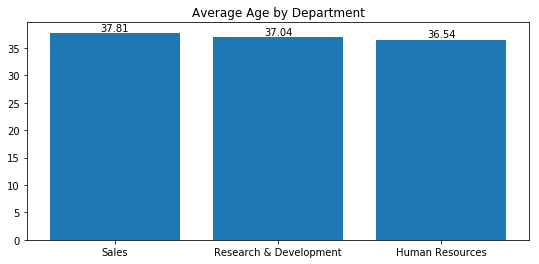This method works for what we've done so far. But the way that we've implemented this so far only works so long as we are completing everything within the single cell.

In :
plt.figure(figsize=(9,4))

Out:
<Figure size 648x288 with 0 Axes>
<Figure size 648x288 with 0 Axes>
In :
bars = plt.bar(empl_data['Department'].unique(),empl_data.groupby('Department')['Age'].mean())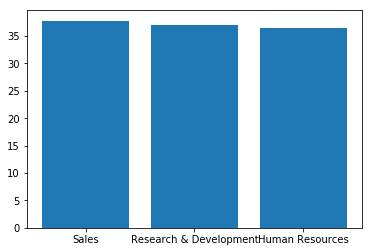When the figure is defined in a separate step, the bars are not placed on a figure size of 9x4. To correct for this, we'll introduce subplots.

In :
f, ax = plt.subplots(1,1,figsize=(9,4))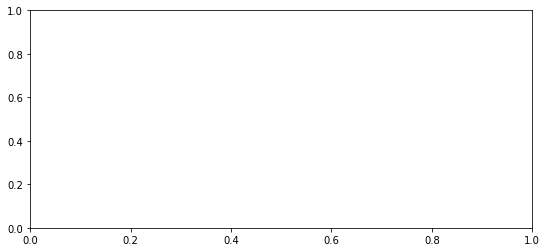In :
ax.bar(empl_data['Department'].unique(),empl_data.groupby('Department')['Age'].mean())

Out:
<BarContainer object of 3 artists>
In :
ax.set_title('Average Age by Department')

Out:
Text(0.5,1,'Average Age by Department')
In :
f

Out:Using a subplot, we were able to continually add and build the plot - we didn't have to complete the entire plot in one step.

Shortly, we'll use subplots to split out three distinct plots for each department, rather than putting all in one plot.

The average does not appear to change across the departments, what about the median age?.

In :
# median employee age by department

plt.figure(figsize=(9,4))
bars = plt.bar(empl_data['Department'].unique(),empl_data.groupby('Department')['Age'].median())

# create a function to calculate and add the labels
def label(bars):
for bar in bars:
height = bar.get_height()
plt.text(bar.get_x() + bar.get_width()/2., 1.0*height,
'%0.2f' % height, va='bottom', ha='center')

label(bars)

plt.title('Median Age by Department')
plt.show()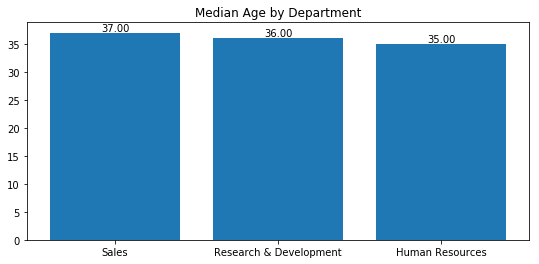Not significant, but we do observe a change in the median age greater than the average age.

Next, let's take a look at the distribution of ages.

In :
# histogram showing distribution of employee ages

plt.figure(figsize=(9,4))
plt.hist(empl_data['Age'])
plt.xlabel('Age')
plt.ylabel('Frequency')
plt.title('Age Distribution of Employees')
plt.grid(False)
plt.show()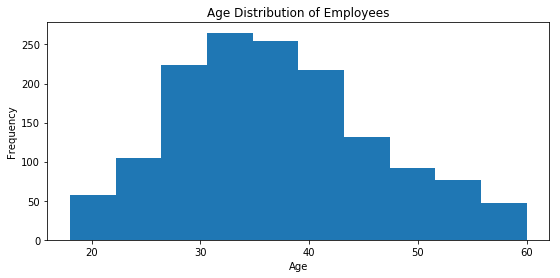In :
# matplotlib allows us to control the number of bins in the histogram

plt.figure(figsize=(9,4))
plt.hist(empl_data['Age'], bins=25)
plt.xlabel('Age')
plt.ylabel('Frequency')
plt.title('Age Distribution of Employees')
plt.grid(False)
plt.show()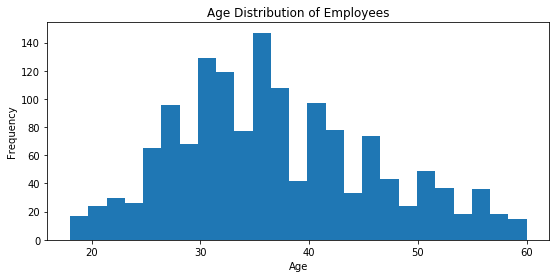In :
# age distribution by department

# create subplots
f, axs = plt.subplots(1,3, sharey='row', figsize=(16,4))

# label each subplot
axs.set_title('Human Resources')
axs.set_title('Sales')
axs.set_title('Research & Development')

# add histogram to each subplot
axs.hist(empl_data[empl_data['Department']=='Human Resources']['Age'], bins=10)
axs.hist(empl_data[empl_data['Department']=='Sales']['Age'], bins=10)
axs.hist(empl_data[empl_data['Department']=='Research & Development']['Age'], bins=10)

# show the plot
plt.show()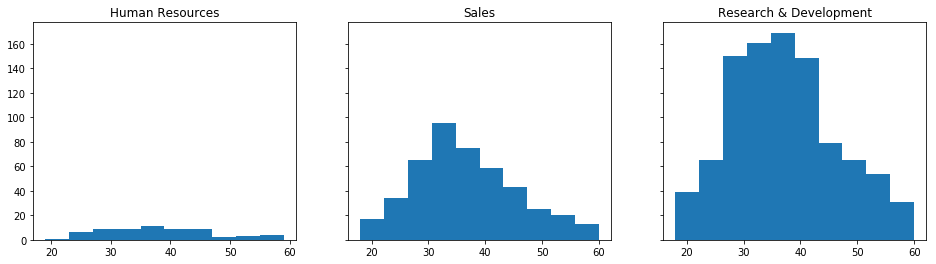In :
# allow each y-axis scale to be independent of other plots

fig = plt.figure(figsize = (16,4))
sub1 = plt.subplot(1,3,1)
sub1.set_title('Human Resources')
sub1.hist(empl_data[empl_data['Department']=='Human Resources']['Age'], bins=10)
sub2 = plt.subplot(1,3,2)
sub2.set_title('Sales')
sub2.hist(empl_data[empl_data['Department']=='Sales']['Age'], bins=10)
sub3 = plt.subplot(1,3,3)
sub3.set_title('Research & Development')
sub3.hist(empl_data[empl_data['Department']=='Research & Development']['Age'], bins=10)

plt.show()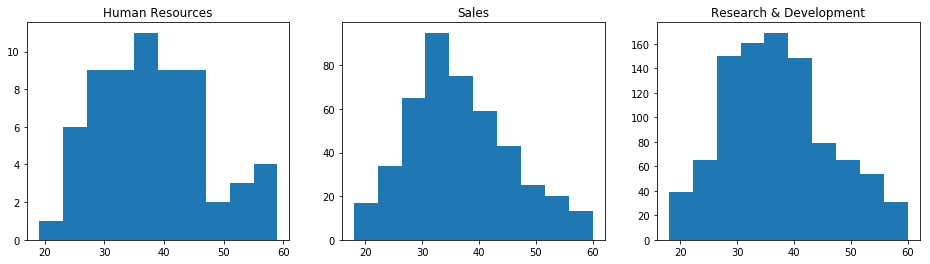In :
# allow each y-axis scale to be independent of other plots

fig = plt.figure(figsize = (16,4))
sub1 = plt.subplot(1,3,1)
sub1.set_title('Human Resources')
sub1.hist(empl_data[empl_data['Department']=='Human Resources']['Age'], bins=10)
sub2 = plt.subplot(1,3,2)
sub2.set_title('Sales')
sub2.hist(empl_data[empl_data['Department']=='Sales']['Age'], bins=10)
sub3 = plt.subplot(1,3,3)
sub3.set_title('Research & Development')
sub3.hist(empl_data[empl_data['Department']=='Research & Development']['Age'], bins=10)

# tight_layout adjusts the scale and layout
plt.tight_layout()
plt.show()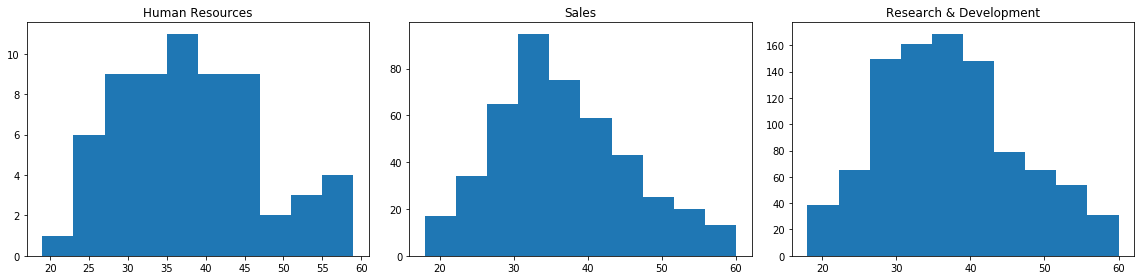In :
# add color

fig = plt.figure(figsize = (16,4))
sub1 = plt.subplot(1,3,1)
sub1.set_title('Human Resources')
sub1.hist(empl_data[empl_data['Department']=='Human Resources']['Age'], bins=10, color='#6b91ce')
sub2 = plt.subplot(1,3,2)
sub2.set_title('Sales')
sub2.hist(empl_data[empl_data['Department']=='Sales']['Age'], bins=10, color='#AA0000')
sub3 = plt.subplot(1,3,3)
sub3.set_title('Research & Development')
sub3.hist(empl_data[empl_data['Department']=='Research & Development']['Age'], bins=10, color='green')
plt.tight_layout()
plt.show()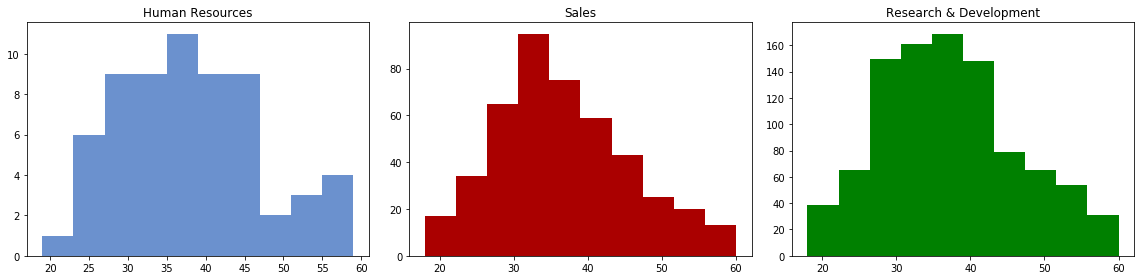Subplots are very powerful, and can be completely controlled independent of one another. You can place any visualization within each subplot.

In :
fig = plt.figure(figsize = (16,4))
sub1 = plt.subplot(1,3,1)
sub1.set_title('Histogram')
sub1.hist(empl_data[empl_data['Department']=='Sales']['Age'], bins=10, color='#6b91ce')
sub2 = plt.subplot(1,3,2)
sub2.set_title('Violinplot')
sub2.violinplot(empl_data[empl_data['Department']=='Sales']['Age'])
sub3 = plt.subplot(1,3,3)
sub3.set_title('Boxplot')
sub3.boxplot(empl_data[empl_data['Department']=='Sales']['Age'])
plt.tight_layout()
plt.show()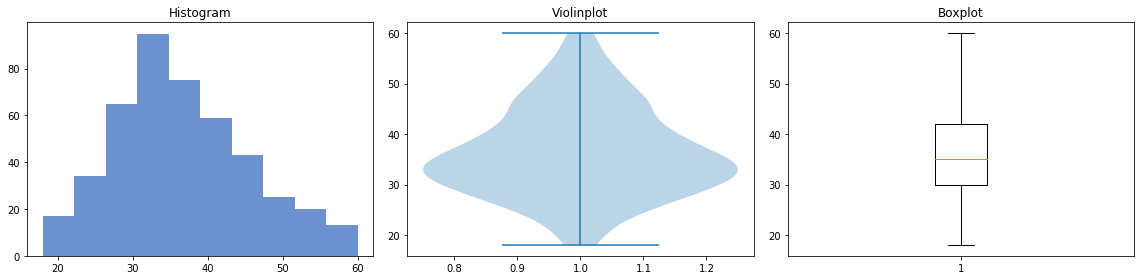### Findings

Having explored Age, we learned:

• The average age is roughly 37 years.
• This average does not change much across the 3 departments.